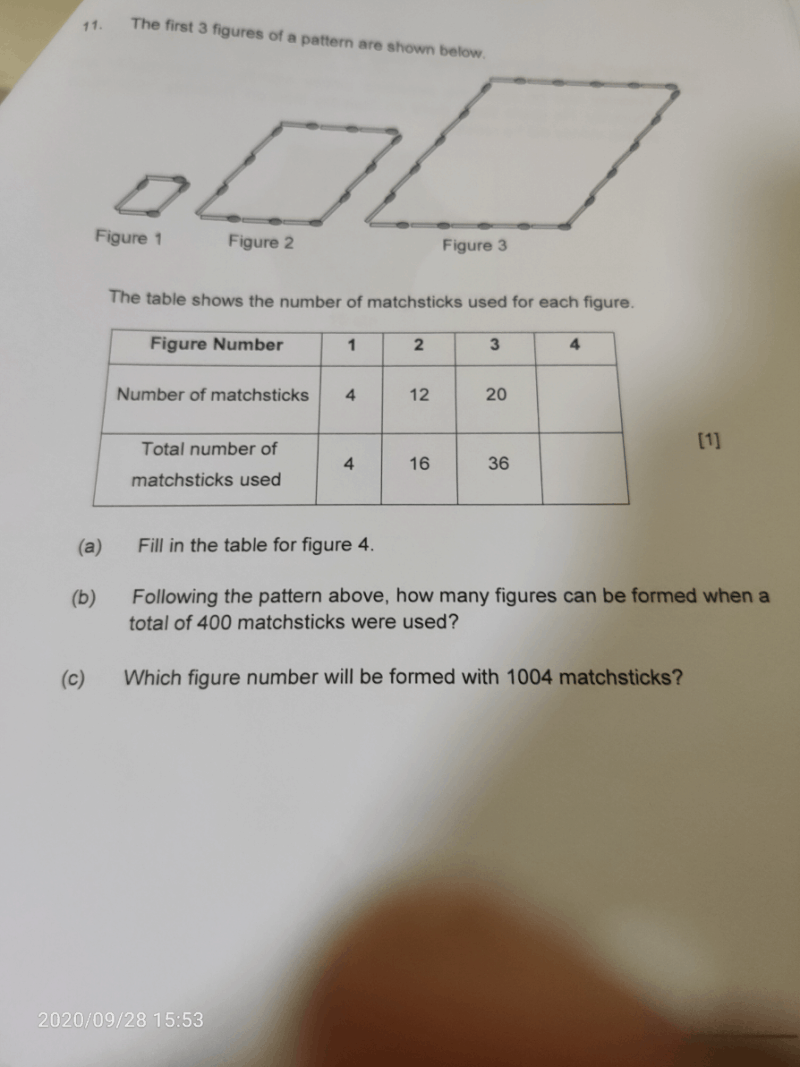# QuestionFormula to find out the no. of matchsticks per figure: (Fig. No. x 2 – 1) x 4

Formula to find out the total no. of matchsticks per figure: (Fig. No. x Fig. No.) x 4

So,

No. of matchsticks for Fig. 4 –> (4 x 2 – 1) x 4 = 28 (a)(i)

Total no. of matchsticks for Fig. 4 –> (4 x 4) x 4 = 64 (a)(ii)

Figures formed for when a total of 400 matchsticks are used –> √(400 ÷ 4) = 10 (b)

Fig. No. formed with 1004 matchsticks –> (1004 ÷ 4 + 1) ÷ 2 = 126 (c)

Hope you understand and all the best with your exams! :))

0 Replies 1 Like